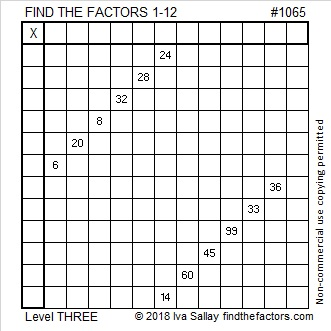# 1065 and Level 3

24 and 14 have two common factors, but just one of them will put only numbers from 1 to 12 in the first column. After you write those factors on the puzzle, work down the first column of the puzzle cell by cell writing the appropriate factors as you go.Print the puzzles or type the solution in this excel file: 12 factors 1063-1072

Here are a few facts about the number 1065:

• 1065 is a composite number.
• Prime factorization: 1065 = 3 × 5 × 71
• The exponents in the prime factorization are 1, 1, and 1. Adding one to each and multiplying we get (1 + 1)(1 + 1)(1 + 1) = 2 × 2 × 2 = 8. Therefore 1065 has exactly 8 factors.
• Factors of 1065: 1, 3, 5, 15, 71, 213, 355, 1065
• Factor pairs: 1065 = 1 × 1065, 3 × 355, 5 × 213, or 15 × 71
• 1065 has no square factors that allow its square root to be simplified. √1065 ≈ 32.634341065 is the hypotenuse of a Pythagorean triple:
639-852-1065 which is (3-4-5) times 213

1065 is a palindrome in two bases:
It’s 353 in BASE 18 because 3(18²) + 5(18) + 3(1) = 1065
1A1 in BASE 28 (A is 10 base 10) because 28² + 10(28) + 1 = 1065

This site uses Akismet to reduce spam. Learn how your comment data is processed.